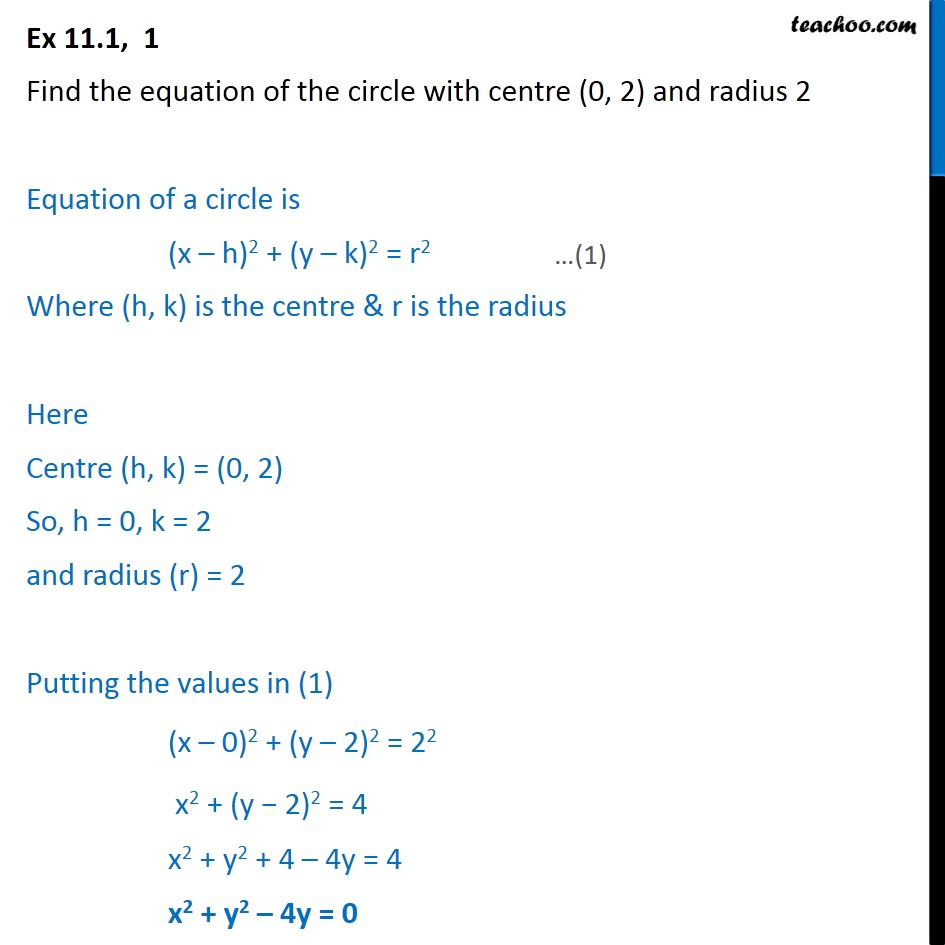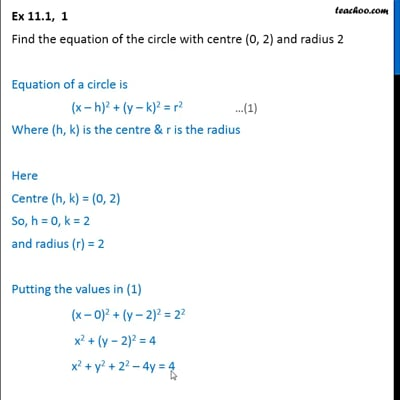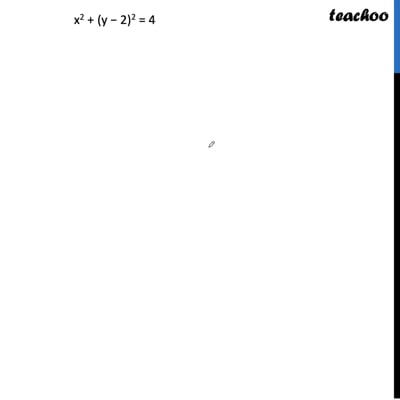Ex 11.1

Chapter 11 Class 11 Conic Sections
Serial order wiseThis video is only available for Teachoo black usersThis video is only available for Teachoo black users

Get live Maths 1-on-1 Classs - Class 6 to 12

### Transcript

Ex 11.1, 1 Find the equation of the circle with centre (0, 2) and radius 2 Equation of a circle is (x h)2 + (y k)2 = r2 Where (h, k) is the centre & r is the radius Here Centre (h, k) = (0, 2) So, h = 0, k = 2 and radius (r) = 2 Putting the values in (1) (x 0)2 + (y 2)2 = 22 x2 + (y 2)2 = 4 x2 + y2 + 4 4y = 4 x2 + y2 4y = 0